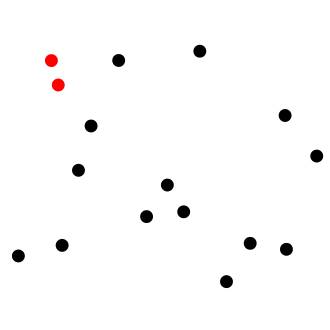Computer Science

# Basic Shapes, Polygons, TrigonometryGiven an array of $n$ points, write an algorithm to compute the closest pair points.

In the figure above the closest pair of points have been marked in red. Given a set of points shown below, let $d$ be the distance between the closest pair of point. Then what is the value of $\left\lfloor 100d \right\rfloor$?

$S=\{(4,0), (6,2), (9,4), (8,9), (1,2), (3,5), (3, 1)\}$

Let the two closest points in the following set of coordinate points be $P_1=(x_1,y_1)$ and $P_2 = (x_2,y_2)$. What is the value of $x_1+y_1+x_2+y_2$?

Given a polygon $P$ and a point $p$ implement an algorithm to check whether the point lies inside the polygon or not.

The algorithm should output $1$ if the point is inside the polygon, and $0$ if it isn't. Consider the following pairs of polygon and point shown below, if $l_{n}$ in the value output by the algorithm for the $n$th pair of polygon and point, what is the value of the string $[l_{1}l_{2}l_{3}l_{4}]$?

$P_1=[(-2, 1), (1, 3), (3, -3)], \\ p_1=(1,1)$

$P_2=[(2, 4), (4, 2), (6, 8), (8,6)], \\ p_2=(3,3)$

$P_3=[(-5,2), (8,2), (8,-4), (-5,-4)], \\ p_3=(-6,1)$

$P_4= [(-2,2), (2,2), (-6,0), (6,0)], \\ p_4=(3,1)$

Details and assumptions

The points lying on the border of the polygon are considering to be inside the polygon

How many of the $24$ triangles below enclose the origin within their perimeter?

  1 2 3 4 5 6 7 8 9 10 11 12 13 14 15 16 17 18 19 20 21 22 23 24 ((86, 74, 44), (38, 47, 68), (48, 92, 81)) ((37, 59, 35), (-84, -71, -23), (57, -71, -34)) ((-16, -22, -71), (-85, -74, 8), (-54, 46, 92)) ((58, -60, 83), (27, -2, 77), (72, -47, 28)) ((62, 17, 89), (-32, 84, 59), (61, -85, -9)) ((8, 80, -80), (8, -73, -30), (40, -6, 36)) ((1, -13, 34), (34, -12, -63), (62, 70, 22)) ((-40, -20, -39), (63, -2, -28), (-86, 13, -60)) ((54, -30, 0), (63, -77, -27), (71, 8, -5)) ((-90, -35, 25), (-64, 22, 36), (-34, -21, 11)) ((57, 54, -96), (-33, 53, 93), (91, -23, 32)) ((-17, -73, -85), (-71, 93, 83), (-36, -59, -48)) ((-49, -29, 18), (-60, 55, 9), (37, -78, -92)) ((-69, 21, 60), (98, -81, -49), (89, 31, -87)) ((-5, -97, -45), (29, 36, -98), (70, -39, -10)) ((-74, 89, -64), (-8, 36, 59), (-5, 4, -29)) ((74, -50, -58), (-13, 60, 61), (98, 4, 99)) ((-25, 75, 55), (84, 29, 34), (-50, -41, 84)) ((93, -97, -88), (-50, 27, 9), (-100, 16, 26)) ((86, -82, 36), (83, -52, -74), (43, 16, 4)) ((-1, -17, 53), (-6, 56, 44), (15, -7, 29)) ((-63, -7, -34), (60, 100, 91), (-13, 20, -65)) ((-33, 64, -18), (28, 5, -81), (-78, 52, 41)) ((21, -20, -76), (51, -85, -28), (-91, 90, 70)) 

×